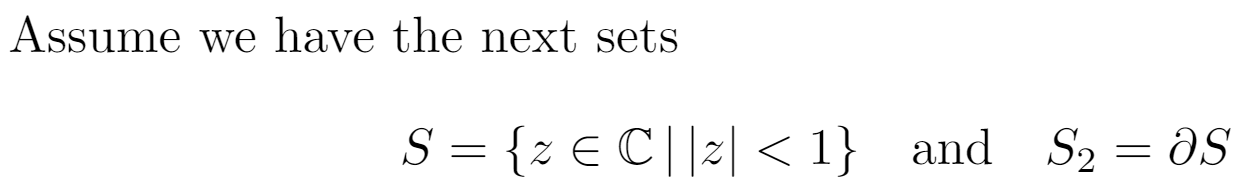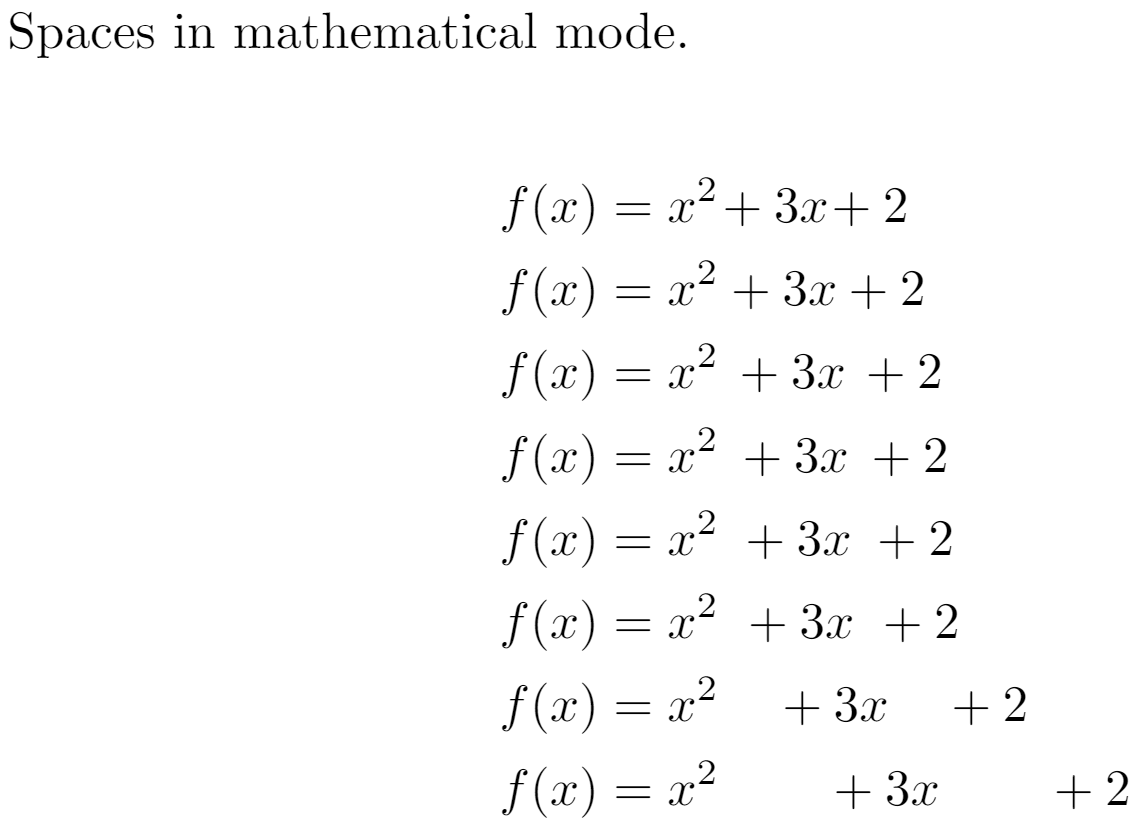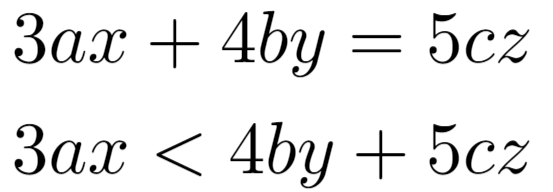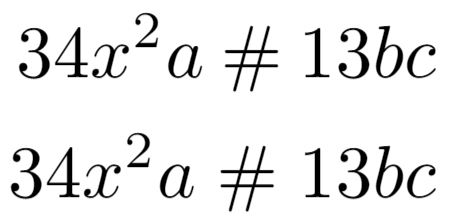This article explains how to insert spaces of different widths in math mode.

# Introduction

Adjusting (La)TeX's default math mode spacing can be useful in certain situations; let's see an example:

\documentclass{article}
\usepackage{amssymb}
\begin{document}
Assume we have the next sets
$S = \{ z \in \mathbb{C}\, |\, |z| < 1 \} \quad \textrm{and} \quad S_2=\partial{S}$
\end{document}


This example produces the following output:As you see in this example, a mathematical text can be explicitly spaced by means of some special commands.

# Spaces

The example below contains a complete list of spaces inserted using various commands and demonstrates their effect on the typeset math.

\documentclass{article}
\usepackage{amsmath}
\begin{document}
Spaces in mathematical mode.

\begin{align*}
f(x) &= x^2\! +3x\! +2 \\
f(x) &= x^2+3x+2 \\
f(x) &= x^2\, +3x\, +2 \\
f(x) &= x^2\: +3x\: +2 \\
f(x) &= x^2\; +3x\; +2 \\
f(x) &= x^2\ +3x\ +2 \\
\end{align*}
\end{document}


This example produces the following output:Check the reference guide for a description of the commands.

Note: to see a description of the align* environment see Aligning equations with amsmath

# Operators spacing

Spacing around operators and relations in math mode are governed by specific skip widths:

• \thinmuskip (by default it is equal to 3 mu)
• \medmuskip (by default it is equal to 4 mu)
• \thickmuskip (by default it is equal to 5 mu)

\begin{align*}
3ax+4by=5cz\\
3ax<4by+5cz
\end{align*}


This example produces the following output:For relational operators, such as $$<$$, $$>$$ and $$=$$, LaTeX establishes \thickmuskip space. But for binary operators such as $$+$$, $$-$$ and $$\times$$, the \medmuskip space is set. The difference is almost unnoticeable.

# User-defined binary and relational operators

You can force the spacing used in binary or relational operators, so you can define your own.

\begin{align*}
34x^2a \mathbin{\#} 13bc \\
34x^2a \mathrel{\#} 13bc
\end{align*}


This example produces the following output:The previous example sets a particular spacing before and after # by using \mathrel (relational) and \mathbin (binary) commands.

# Reference guide

Description of spacing commands

LaTeX code Description
\quad space equal to the current font size (= 18 mu)
\, 3/18 of \quad (= 3 mu)
\: 4/18 of \quad (= 4 mu)
\; 5/18 of \quad (= 5 mu)
\! -3/18 of \quad (= -3 mu)
\  (space after backslash!) equivalent of space in normal text
\qquad twice of \quad (= 36 mu)